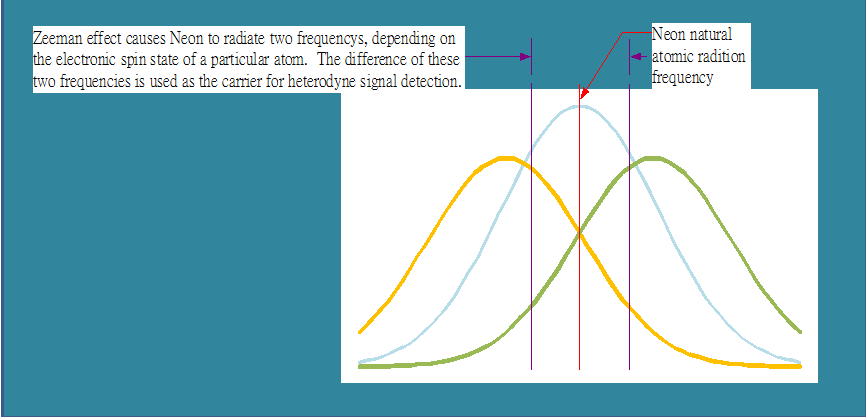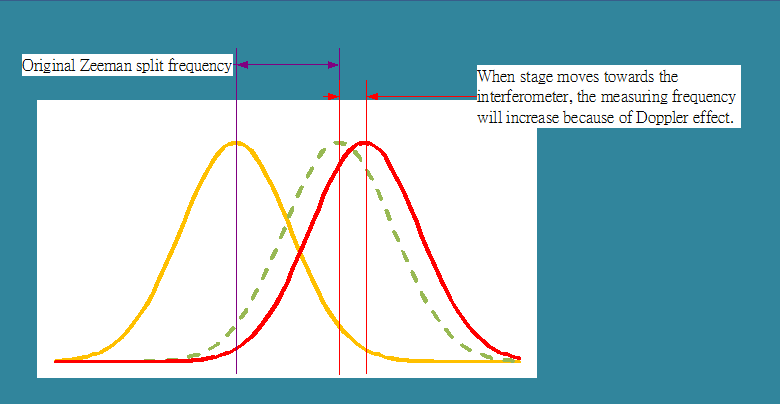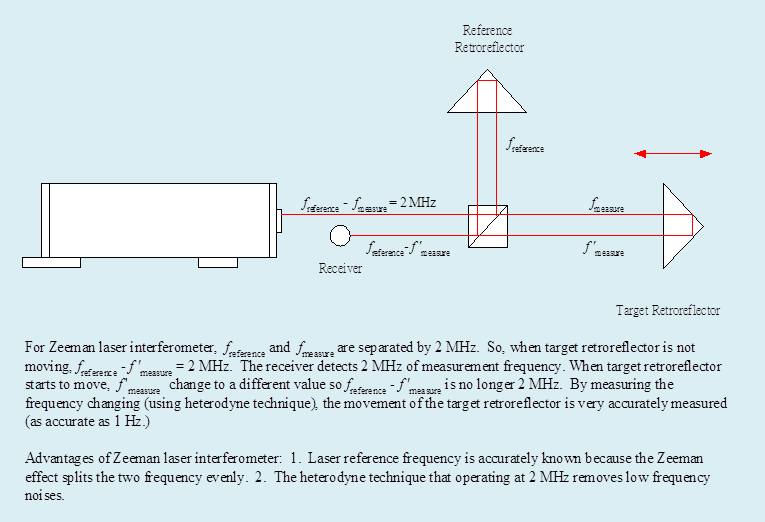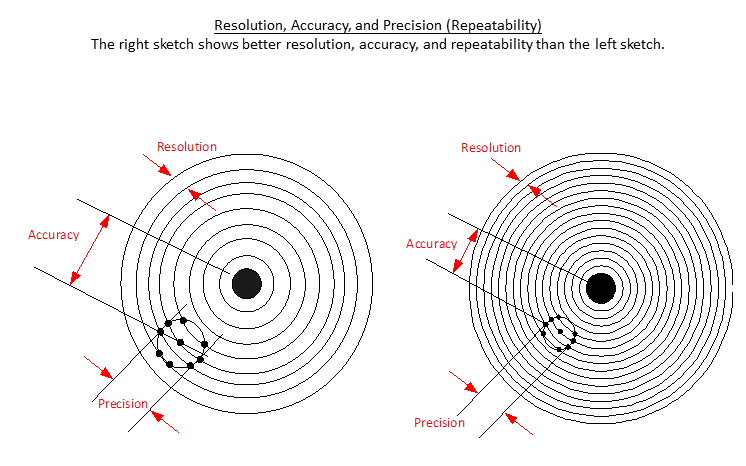# Application Note

## Method of Measurement for the Excel Precision Laser Metrology System

The Excel Model 1000A Laser Position Measurement System is a modern version of the interferometer technique pioneered by A.A. Michelson.

This interferometer technique consists of splitting a monochromatic light beam into two paths and reflecting both paths back to a point in space where they converge. If there is movement of at least one reflector relative to the other, the light from the two beams will interfere one to the other and produce varying intensities at the point where they meet. Each half wave length of reflector travel means a total optical path change of one wavelength and one complete cycle of intensity change. If the wavelength of light is known, the travel of the reflector can be accurately determined. An excellent source of monochromatic light for an interferometer is the light from a laser source.

The Excel Model 1001 Laser Head series is the latest and best source of light for precise interferometer measurements. The Laser Head emits a laser beam consisting of two frequencies: fs and fp. These frequencies are linearly polarized and orthogonal to each other, and parallel and orthogonal to the plane of the feet of the Laser Head.

These two frequencies are produced by the Zeeman splitting of a single longitudinal mode frequency of the He-Ne laser beam by applying an axial magnetic field to the lasing gas.A small portion of the two-frequency beam is used internally in the laser head to provide a feedback signal to stabilize the laser cavity length, and to provide the reference frequency: fs and fp.

The main portion of the beam is used externally as the signal for the interferometer. The interferometer uses a polarizing coating to split the beam into its two frequency components, with one frequency being reflected to a reference reflector and the other frequency being passed to a measurement reflector. This split occurs since the polarizing coating allows the passage of the vertically polarized frequency, but reflects the horizontally polarized frequency.

The two reflectors return their respective parts of the beam to a common coaxial path, then recombine and interfere at the photo-detector of the receivers.

Relative motion between the reference reflector and the measurement reflector causes a Doppler shift in the difference frequency detected by the photodiode. This Doppler shifted frequency becomes the measurement frequency: f – fp, fp.The measurement signal is converted from a light signal to an electrical signal and amplified by the receiver. The measurementsignal output from the receiver and the reference signal output from the Laser Head are both input into the Excel Model 1065 Signal Process. The Signal Processor multiplies the frequency for resolution extension, counts the frequency of both the measurement and reference signals, then makes a frequency comparison. The Excel 1065 Signal Processor then outputs the instantaneous position for motion control circuitry to use.## Required Characteristics of Measuring Instruments

Below are parameters that are used to gauge the quality of a measurement: accuracy, repeatability (precision), and resolution.

• Accuracy – how closely the measured value agrees with the true value
• Precision – a measure of the repeatability of the measurement process
• Rule of 10 : the measuring instrument must be ten times more precise than the specified tolerance
• Resolution – the smallest variation of the variable that can be detected
• Speed of response – how long the instrument takes to measure the variable, i.e. real time or delayed
• Actual measurement or interpolation
• Others: operating range, reliability, cost

The sketch below explains the definitions of accuracy, repeatability, and resolution.